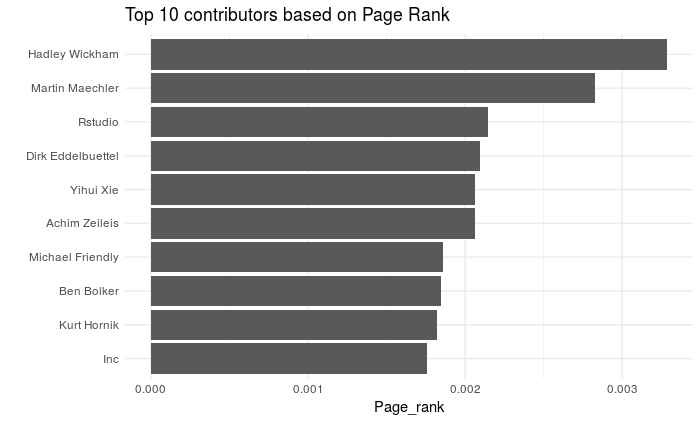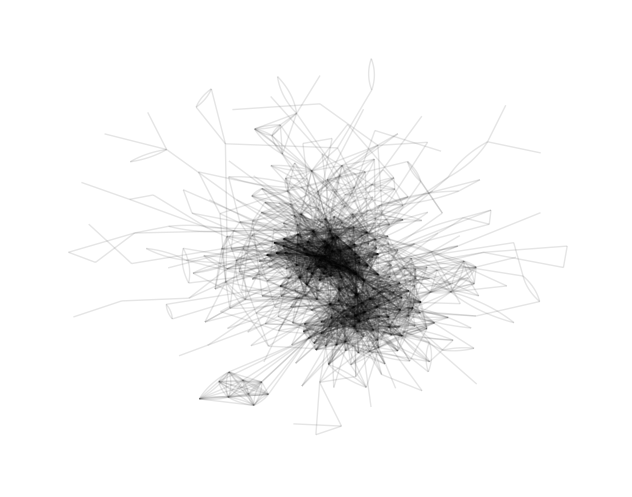Want to share your content on R-bloggers? click here if you have a blog, or here if you don't.

A few months ago, I published a post where I was trying to map the dependencies relationships between R packages. Today I want to do something similar with package contributors. My idea is to reconstruct a social graph where each node would be a person (presumably a developer), and two persons would be connected by an edge if they have collaborated on the same package. Thus I would be able to explore the CRAN social network!

This post is also a bit special for me. It’s the very first time I’m using `dplyr` and the tidyverse. I used to write my code in base R but the amazing work of Thomas Lin Pedersen around `tiygraph` and `ggraph` convinced me to take the plunge. And it was a lot of fun!

First, we load the packages and the data. In the first version of the project I used the XML file of each package that I retrieved from CRAN using wget and then read and parsed with `xml2`. Since `R 3.4.0`, things are much, much simpler with the function `CRAN_package_db`.

```library(tidyverse)
library(stringr)
library(igraph)
library(tidygraph)
library(ggraph)
library(magrittr)

pdb <- tools::CRAN_package_db()
aut <- pdb\$Author
```

That’s all we need to get the full list of contributors. But it comes in a very messy way. R provides a structured system to describe persons which should be used in the `Authors@R` field of the DESCRIPTION file, but most people use a simple character string.

# The big cleanup

I combined a series of regular expressions and string manipulations to extract every name. It was the first time I was using `stringr` and I have to say, I don’t regret `gsub`, `grepl`, etc. I just miss the support for some PCRE functionalities…

```aut <- aut %>%
str_replace_all("\\(([^)]+)\\)", "") %>%
str_replace_all("\\[([^]]+)\\]", "") %>%
str_replace_all("<([^>]+)>", "") %>%
str_replace_all("\n", " ") %>%
str_replace_all("[Cc]ontribution.* from|[Cc]ontribution.* by|[Cc]ontributors", " ") %>%
str_replace_all("\\(|\\)|\\[|\\]", " ") %>%
iconv(to = "ASCII//TRANSLIT") %>%
str_replace_all("'\$|^'", "") %>%
gsub("([A-Z])([A-Z]{1,})", "\\1\\L\\2", ., perl = TRUE) %>%
gsub("\\b([A-Z]{1}) \\b", "\\1\\. ", .) %>%
map(str_split, ",|;|&| \\. |--|(?<=[a-z])\\.| [Aa]nd | [Ww]ith | [Bb]y ", simplify = TRUE) %>%
map(str_replace_all, "[[:space:]]+", " ") %>%
map(str_replace_all, " \$|^ | \\.", "") %>%
map(function(x) x[str_length(x) != 0]) %>%
set_names(pdb\$Package) %>%
extract(map_lgl(., function(x) length(x) > 1))

aut_list <- aut %>%
unlist() %>%
dplyr::as_data_frame() %>%
count(value) %>%
rename(Name = value, Package = n)
```

At this point we have a list of 13668 (unique) names, and this number is increasing almost every day. The R community is huge! Of course there are still some errors. And we can find surprising contributors:

```aut_list\$Name

#  "Her Majesty the Queen in Right of Canada"
```

# The Social Network

The next step is to produce a two-column matrix describing all the connections of the network (edge list). An edge list can be turned into a graph object with the `igraph` package. Finally we can convert the igraph graph into a tidy graph so we can use the API provided by the `tidygraph` package. For example to filter nodes/edges or select only the main component.

```edge_list <- aut %>%
map(combn, m = 2) %>%
do.call("cbind", .) %>%
t() %>%
dplyr::as_data_frame() %>%
arrange(V1, V2) %>%
count(V1, V2)

g <- edge_list %>%
select(V1, V2) %>%
as.matrix() %>%
graph.edgelist(directed = FALSE) %>%
as_tbl_graph() %>%
activate("edges") %>%
mutate(Weight = edge_list\$n) %>%
activate("nodes") %>%
rename(Name = name) %>%
mutate(Component = group_components()) %>%
filter(Component == names(table(Component))[which.max(table(Component))])
```

The author of `tidygraph`, Thomas, also developed the `ggraph` package, an implementation of the grammar of graphics for relational data. Using `ggraph`, it is very easy to visualize our graph.

```ggraph(g, layout = 'lgl') +
geom_edge_fan(alpha = 0.1) +
theme_graph()
```This is it! This hairy ink stain is the main connected component of the CRAN social network, representing collaborations between 6758 developers!

We can look for who’s the « most important » node in the graph according to the Google’s PageRank algorithm, although it’s not straightforward what could mean a high PR here…

```g %>%
mutate(Page_rank = centrality_pagerank()) %>%
top_n(10, Page_rank) %>%
as_tibble() %>%
ggplot() +
geom_col(aes(forcats::fct_reorder(Name, Page_rank), Page_rank)) +
coord_flip() + theme_minimal() +
labs(title = "Top 10 contributors based on Page Rank", x = "")
```# Focus on the core network

The complete graph makes a nice painting to hang on the wall of your living room. But hard to say anything about it. So, we will reduce the data and focus on the contributors involved in more than 4 packages.

```g <- g %>%
left_join(aut_list) %>%
filter(Package > 4) %>%
mutate(Component = group_components()) %>%
filter(Component == names(table(Component))[which.max(table(Component))])

ggraph(g, layout = 'lgl') +
geom_edge_fan(alpha = 0.1) +
theme_graph()
```Nice! It seems like there are two distinct clusters in the middle. Let’s see if we can identify them using a community detection algorithm.

```g <- mutate(g, Community = group_edge_betweenness(),
Degree = centrality_degree())

filter(g, Community == names(sort(table(Community), decr = TRUE))) %>%
select(Name, Package) %>%
arrange(desc(Package)) %>%
top_n(10, Package) %>%
as_tibble() %>%
knitr::kable(format = "html", caption = "Cluster 1")
filter(g, Community == names(sort(table(Community), decr = TRUE))) %>%
select(Name, Package) %>%
arrange(desc(Package)) %>%
top_n(10, Package) %>%
as_tibble() %>%
knitr::kable(format = "html", caption = "Cluster 2")
```
Cluster 1
Name Package
Kurt Hornik 56
Martin Maechler 46
Achim Zeileis 44
Dirk Eddelbuettel 41
Romain Francois 27
Ben Bolker 25
Brian Ripley 25
Torsten Hothorn 24
Roger Bivand 23
Douglas Bates 23
Cluster 2
Name Package
Rstudio 92
Scott Chamberlain 44
Inc 36
Yihui Xie 34
Jeroen Ooms 33
Jj Allaire 29
R. Core Team 27
Bob Rudis 26
Gabor Csardi 22
Oliver Keyes 22
Winston Chang 22

If you know a bit R and its community, you will certainly recognize some names. The challenge is to interpret this classification. It is not a good thing to put people in boxes, but this is mandatory to make nice and colorful visualizations! So, I give it a try. For me, the first group include people of the early days who contributed to major packages which constitute the heart of R. The second group is more related to the second generation, associated with Rstudio products and who have been particularly prolific in the last years. Of course the two groups are strongly connected.

How can we label these two groups? After long consideration, I chose to go for « The Ancients » and « The Moderns », without any value judgment! This is a reference to an old debate in French literature. This dubious comparison would certainly be more appropriate to designate the ongoing debate base vs. tidyverse, but… not today!

Let’s see how we can visualize these two groups.

```g <- g %>%
mutate(Community = case_when(Community == names(sort(table(Community),
decr = TRUE)) ~ "The Ancients",
Community == names(sort(table(Community),
decr = TRUE)) ~ "The Moderns",
Community %in% names(sort(table(Community),
decr = TRUE))[-1:-2] ~ "Unclassified")) %>%
mutate(Community = factor(Community))

g <- g %>%
filter(Degree > 5) %>%
mutate(Degree = centrality_degree())

ggraph(g, layout = 'lgl') +
geom_edge_fan(alpha = 0.1) +
geom_node_point(aes(color = Community, size = Package)) +
theme_graph() +
scale_color_manual(breaks = c("The Ancients", "The Moderns"),
values=c("#F8766D", "#00BFC4", "#969696"))
````ggraph` really does a great job! I really like the result.

BTW, the R community is not really divided…

```g %>% activate("edges") %>%
mutate(Community_from = .N()\$Community[from],
Community_to = .N()\$Community[to]) %>%
filter((Community_from == "The Ancients" & Community_to == "The Moderns")|
Community_from == "The Moderns" & Community_to == "The Ancients") %>%
as_tibble() %>%
nrow()
```

… there are 254 edges connecting the the Ancients and the Moderns! But I think this is a nice illustration of how social graphs keeps the imprint of history and past events. Next step would be to look at the graph dynamics over time. Maybe an idea for a future post…